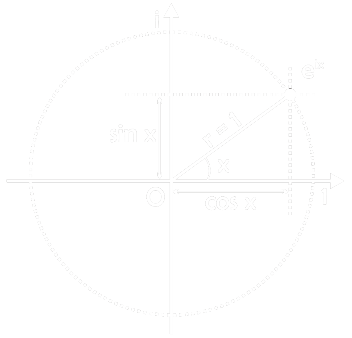Home > Math Shortcuts > Math Euler’s Formula

# Math Euler’s Formula

Let prime p and any a∈Z so that a ≡/0 mod p
ap-1 ≡ 1 mod p.• eix = cos x + i sin x• e = cos π + i sin π
• e = – 1 + i x 0
• e = – 1
• e + 1 = 0

• ez = ex+iy = ex (cos y + i sin y)